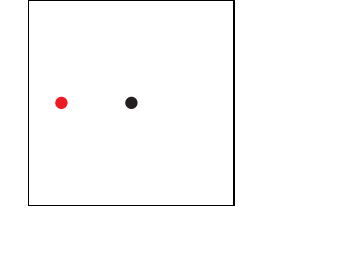## Robert Harlander

 phone: +49-241-80-27045 fax: +49-241-80-22187 harlander(at)physik.rwth-aachen.de Büro: 28A414, Campus Melaten

Home

Brief CV

Research

Teaching

Outreach

Conferences

– Software –

Press

collaborations:

LHCHWG

LHC and Philosophy

P3H

DFG RTG

[Up: aximate] aximate: eom

## Equations of motion

(thanks to contributions from Tim Kempkens, 2019)
 While the evolve feature determines the movement of an object according to an explicit function of the "time" \$t, you may also specify a differential equation, an "equation of motion" with a set of initial conditions. aximate will numerically solve this equation and move the object accordingly. So far, this works only for the coordinates of a point object though. A simple example is given as follows: ``` sub myeom { # This defines the equation of motion in (x,y). # We assume it to be of second order in the time t. my(\$dxdt,\$dydt,\$dvxdt,\$dvydt,@y); my(\$t,\$x,\$y,\$vx,\$vy) = @_; # Defining dx/dt=vx and dy/dt=vy rewrites the eom into a # first-order system of differential equations for (x,y,vx,vy): \$dxdt = \$vx; \$dydt = \$vy; # a parameter: \$g = 100; # The actual differential equation. Try your own here! \$dvxdt = -\$g*\$x/(\$x**2+\$y**2)**(3/2); \$dvydt = -\$g*\$y/(\$x**2+\$y**2)**(3/2); # The output format is required to be this: @y = (\$dxdt,\$dydt,\$dvxdt,\$dvydt); return(@y); } sub manual19 { setparams({"steps" => 100, # number of frames "circular" => 1, # 1: first=last frame "size" => "(200,200)(-100,-100)", "fbox" => 1, "starttime" => 0, "endtime" => 170, "scale" => 1, "loop" => 0 # run to end, then backwards }); # This just marks the center of gravity: point({"coordinates" => [[0,0]]}); # The initial conditions are x(0)=-70, y(0)=0, # vx(0)=0, vy(0)=0.7. # "eomname" is an arbitrary but unique label for the eom. point({"coordinates" => [[-70,0]], "color" => "Red", "eom" => ["eomname",\&myeom], "initv" => [0,.7] }); } ```main
aximate:eom (Robert Harlander)